# Shapes of molecules

The shape of a molecule is determined by the number of electron pairs in its outside shell and whether these electron pairs are bonding or non-bonding.

The Valence Shell Electron Pair Repulsion (VSEPR) theory is used to explain this.

Think only about the central atom of a molecule eg the C of CH4.

Spread the electron pairs of its outside shell around the surface of a sphere. They should be spread out as far as possible.

Since non-bonding electron pairs are held closer to the central atom than the bonding pairs, the non-bonding electron pairs repel the other electron pairs more than bonding electron pairs do.

The way this works is best shown by examples. Check your A-level specification to find out which shapes you have to KNOW and which ones you need to be able to WORK OUT.Start with two electron pairs, both bonding (eg BeCl2). The electrons spread to opposite sides of the imaginary sphere and so the molecule is LINEAR with a bond angle of 180o.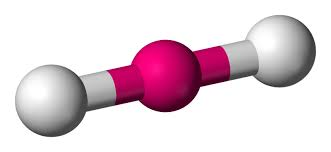A third electron pair (eg BF3) gives a TRIGONAL PLANAR molecule with bond angles of 120o.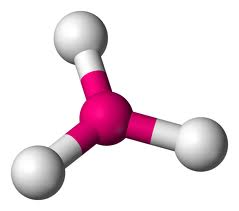Four electron pairs might be thought to give a “X-shaped” arrangement but it turns out that a three-dimensional arrangement works better. The electron pairs point to the corners of a regular tetrahedron. A tetrahedron is a pyramid-like solid with each of its four faces being an equilateral triangle.

If the electron pairs are all bonding, then the shape is TETRAHEDRAL with a bond angle of 109o28′.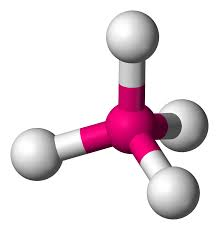If one of the electron pairs is non-bonding, then the shape (defined by the positions of the atoms rather than where the electron pairs are pointing) is known as PYRAMIDAL. The bond angles are approximately 107o for ammonia. The reduction from the tetrahedral angle is because of the extra repulsion of the non-bonding electron pair.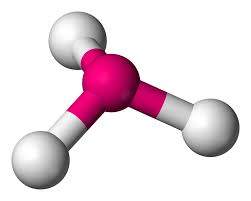If two of the electron pairs are non-bonding, then the shape is known as BENT (or ANGULAR or DIHEDRAL). The bond angles are approximately 104.5o for water. The even-greater reduction from the tetrahedral angle is because of the extra repulsion of two non-bonding electron pairs.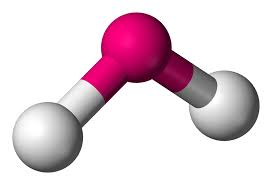Five electron pairs leads to a TRIGONAL BIPYRAMIDAL structure if they are all bonding (eg PF5).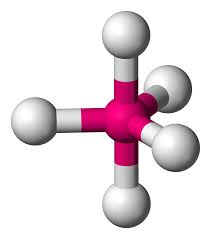Six electron pairs gives an OCTAHEDRAL structure if they are all bonding pairs (eg SF6).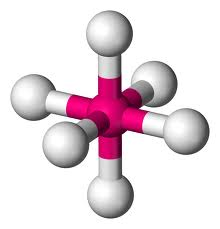but if two of the pairs are non-bonding, a SQUARE PLANAR shape is produced (eg XeF4).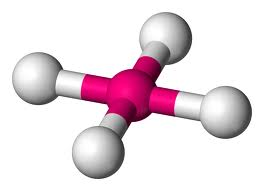THE VIDEO BELOW DETAILS THE SHAPES OF MOLECULES

# Molecular Polarity

The overall polarity of molecules with more than one bond is determined from both the polarity of the individual bonds and the shape of the molecule. Each bond’s dipole moment can be treated as a vector quantity, having a magnitude and direction. Therefore the molecular polarity is the vector sum of the individual bond dipoles.

### How to determine the vector sum

One method to determine the vector sum of dipole arrows is known as the tail-to-head method. Let’s examine this method for a molecule of water. 1. First draw the Lewis electron dot diagram for water and determine its molecular shape. Water has four electron groups, but only two atoms attached to the central atom so it is bent.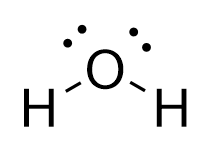2. Draw in dipole arrows for all polar covalent bonds, starting the arrow at the more electropositive atom, and ending at the more electronegative atom.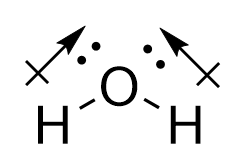3. Connect the dipole arrows tail-to-head.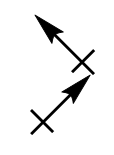4. Draw a new line connecting the tail of the first vector. This is the net molecular dipole.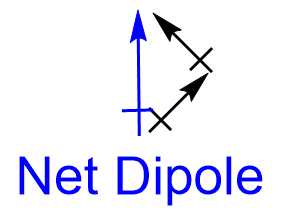5. Now superimpose the net molecular dipole arrow onto the molecule.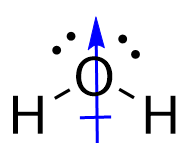An alternative method to determine the vector sum of dipole arrows is known as the vector component method. Let’s examine this method again for a molecule of water. The first two steps remain the same as the tail-to-head method: 1. First draw the Lewis electron dot diagram for water and determine its molecular shape. Water has four electron groups, but only two atoms attached to the central atom so it is bent.

2. Draw in dipole arrows for all polar covalent bonds, starting the arrow at the more electropositive atom, and ending at the more electronegative atom.

3. For dipole arrows at an angle, separate them into horizontal and vertical vector components.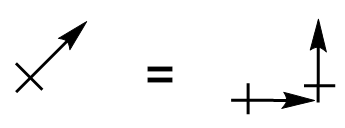4. Superimpose the vector components onto the molecule.
5. Cancel out any vector components that are of equal magnitude and pointing in the opposite direction.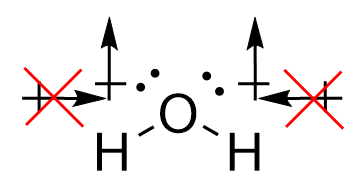As a result of the vector quantity nature of bond dipoles, some molecules may contain polar bonds, yet have no net molecular dipole moment. For example, CO2:

THE VIDEO BELOW TEACHES MORE ABOUT MOLECULAR POLARITY

## STEM Elearning

We at FAWE have built this platform to aid learners, trainers and mentors get practical help with content, an interactive platform and tools to power their teaching and learning of STEM subjects, more